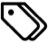Matlab | research | numerical analysis

I just wanted to jot down a few points about Matlab programming. Specifically, this is about finding a string within another cell array of strings, where the thing I’m really interested in is the index of the cell array where the reference string occurs. For example, if my reference string is `'Gamma'`, and my cell array is `{'Alpha','Beta','Gamma','Delta'}`, then the result of the code should be `3`.

Say,

```cellArray = {'Alpha','Beta','Gamma','Delta','GammaSquared'};
refString = 'Gamma';
```

## Method 1

This method uses the Matlab function `strfind` (link).

```index = strfind(cellArray,refString);
index = find(~cellfun(@isempty,index));
```

Result:

```index =
3   5
```

This method works great if the idea is to find a substring, i.e. in the case where we are looking for all possible matches. It doesn’t work too well, however, if we’re looking for a specific match.

## Method 2

This uses the Matlab function `ismember` (link).

```index = find(ismember(cellArray,refString));
```

Result:

```index =
3
```

Works great if the idea is to find a perfect match. However, let’s also keep tabs on the computation time.

```tic; index = find(ismember(cellArray,refString)); toc;
```

Result:

```Elapsed time is 0.001047 seconds.
```

## Method 3

This uses the Matlab function `strcmp` (link).

```index = find(strcmp(cellArray,refString));
```

Result:

```index =
3
```

Same result as in Method 2, but what about computation time?

```tic; index = find(strcmp(cellArray,refString)); toc;
```

Result:

```Elapsed time is 0.000025 seconds.
```

Turns out Method 3 is more than 41 times faster to execute. So we have a winner!

## Reference

Stack Overflow: How to search for a string in cell array in MATLAB?